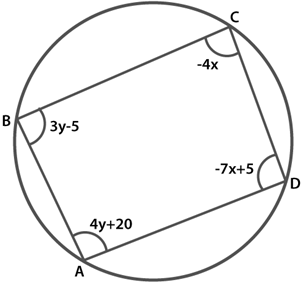# ABCD is a cyclic quadrilateral (see Fig.) . Find the angles of the cyclic quadrilateral.

We have to find the angles of the given quadrilateralWe know that the sum of the opposite angles of a cyclic quadrilateral is 180o

Hence

∠C +∠A = 180

4y + 20− 4x = 180

− 4x + 4y = 160

x − y = − 40 ……………(1)

And, ∠B + ∠D = 180

3y − 5 − 7x + 5 = 180

− 7x + 3y = 180 ………..(2)

On multiplying 3 to equation (1), we get

3x − 3y = − 120 ………(3)

Adding equation (2) to equation (3), we get

− 7x + 3x = 180 – 120

− 4x = 60

x = −15

Substituting this value in equation (i), we get

x − y = − 40

-y−15 = − 40

y = 40-15

= 25

∠A = 4y + 20 = 20+4(25) = 120°

∠B = 3y − 5 = − 5+3(25) = 70°

∠C = − 4x = − 4(− 15) = 60°

∠D = 5-7x

∠D= 5− 7(−15) = 110°

Hence, The angles of quadrilateral are

∠A = 120°

∠B = 70°

∠C= 60°

∠D= 110°